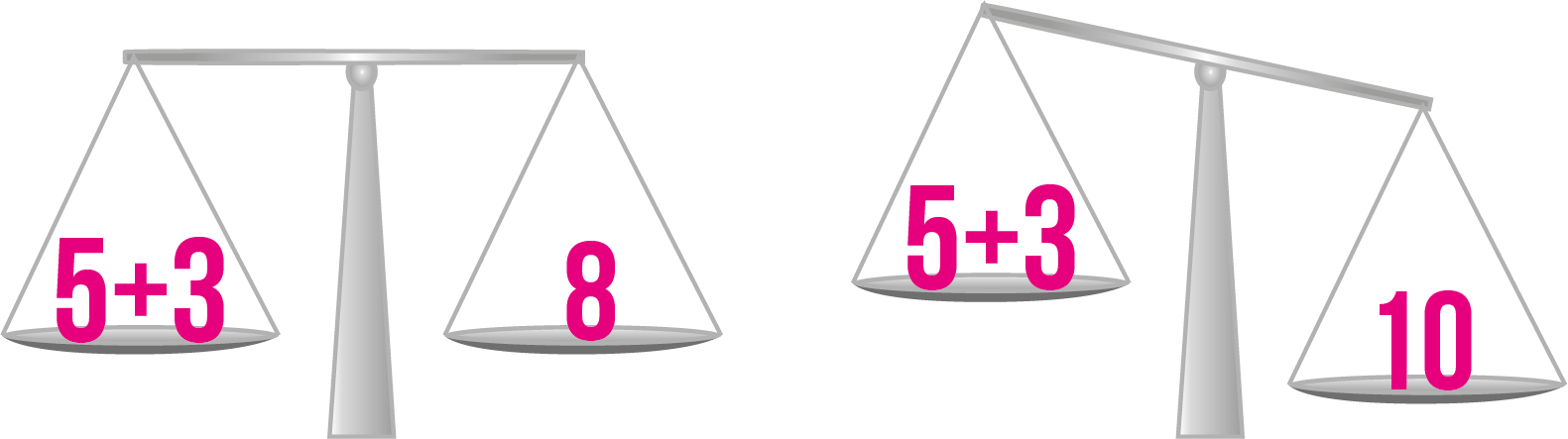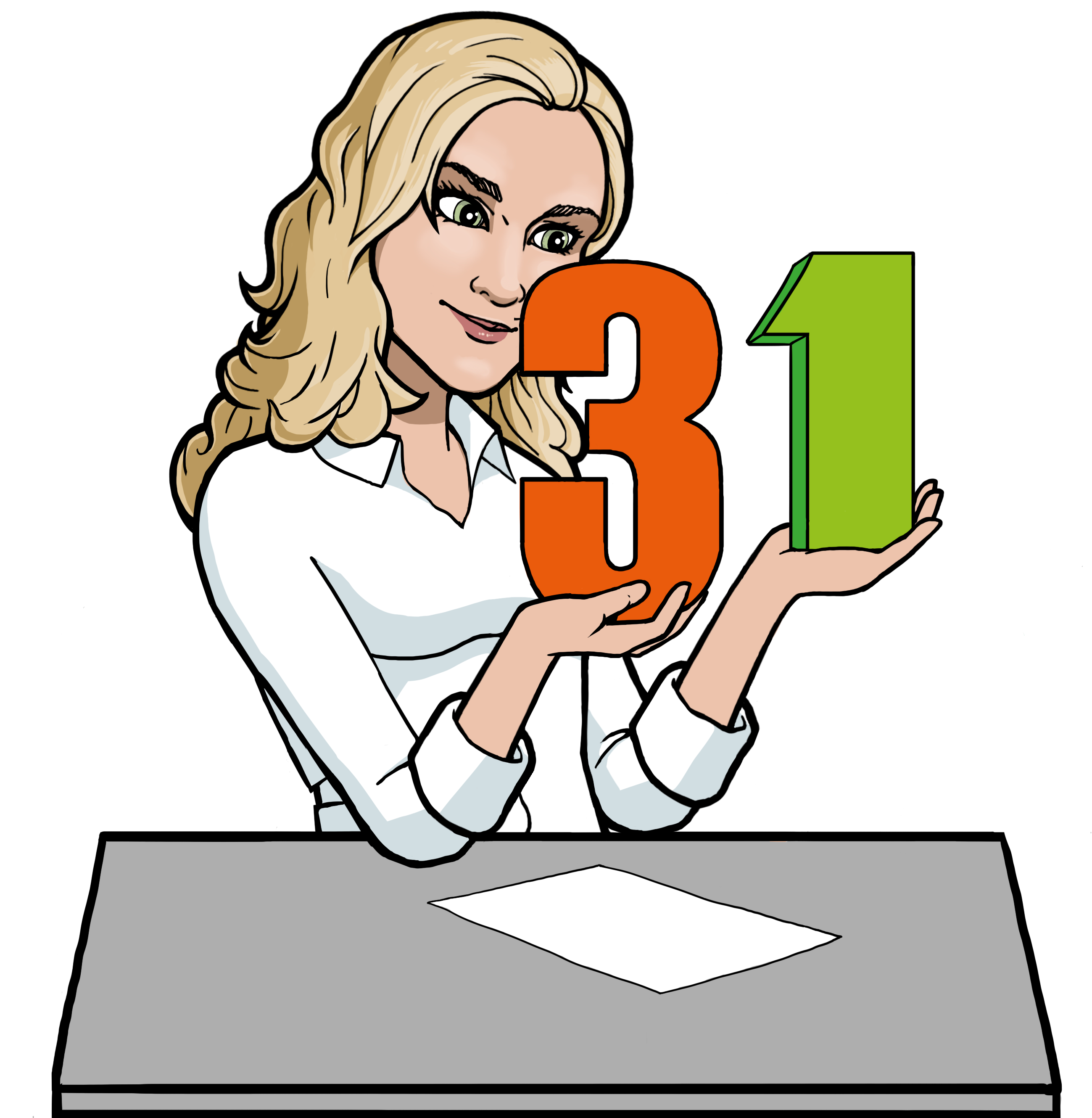# What Is a Variable?

Because mathematics is a language, it’s really important to learn the vocabulary in order to be able to “speak it.” When you’re working with equations, you need to learn the what a variable is, and the change side, change sign rule.

## Variable

In mathematics, variables are written as letters. The reason for this is that the value of a variable can vary, so it wouldn’t make sense to assign numbers to them right away.

In an equation, the right-hand side (RHS) and the left-hand side (LHS) will always be equal to each other. That’s why when you do something on one of the sides, you have to do the same on the other side as well, or the scales will become unbalanced.But what does it mean to “do something” to an equation? The first things you shall learn to do with equations is use the change side, change sign rule. You’ll also learn how to get rid of numbers in front of the variable $x$.

The goal of solving an equation is to find the value of $x$.
You find the value of $x$ by getting it to stand alone on one side of the equation. “Change side, change sign” and “getting rid of numbers in front of $x$” are rules you can apply to isolate $x$. Isolating $x$ means that $x$ is alone on one side of the equation,